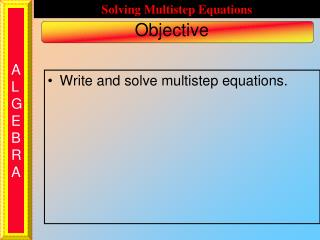DownloadDownload PresentationObjective

# Objective

Télécharger la présentation## Objective

- - - - - - - - - - - - - - - - - - - - - - - - - - - E N D - - - - - - - - - - - - - - - - - - - - - - - - - - -
##### Presentation Transcript

1. Write and solve multistep equations. Objective

2. Key Skills Keep it balanced! Where do we start?Does it matter?Like driving to Seaside Heights, you could take the Turnpike to the Parkway or Route 280 to the Parkway. Either way gets you to the Parkway which you need to take to get to Seaside Heights. Solve 6n + 17 = 5 + 4n Subtract 6n from each side of the equal sign. The goal is to get the variable on one side of the equal sign and the numbers on the other side. – 6n – 6n Subtract 5 from each side of the equal sign. 17 = 5 – 2n –5 –5 Divide by – 2 12 = – 2n n = – 6 ÷ – 2 ÷ – 2

3. Key Skills Keep it balanced! Solve 3x + 19 = 4 + 8x Subtract 3x from each side of the equal sign. The goal is to get the variable on one side of the equal sign and the numbers on the other side. – 3x – 3x Subtract 4 from each side of the equal sign. 19 = 4 + 5x –4–4 Divide by 5 15 = 5x x = 3 ÷ 5 ÷ 5

4. Try This Keep it balanced! Solve – 3x – 9 = – 13 – 7x Add 3x to each side of the equal sign. The goal is to get the variable on one side of the equal sign and the numbers on the other side. + 3x + 3x Add 13 to each side of the equal sign. – 9 = –13 – 4x +13 +13 Divide by – 4 4 = – 4x x = – 1 ÷ – 4 ÷ – 4

5. Do this together Mr. Esker has to buy pencils for his company. Supplier A charges \$4 per box and \$3 for shipping. Supplier B charges \$3 per box and \$6 for shipping. a. Write equations for both suppliers. b. How many boxes would he need to buy for both companies to charge the same amount?

6. Do this together Mr. Esker has to buy pencils for his company. Supplier A charges \$4 per box and \$3 for shipping. Supplier B charges \$3 per box and \$6 for shipping. To write the equation for Supplier A, the Cost (what you end up paying) is equal to + 4b C = \$3 the shipping cost (fixed amount, the constant where the cost starts) + the cost per box multiplied by how boxes are purchased. Try writing the equation for Supplier B. C = \$6 + 3b

7. Do this together In order to find when the 2 Suppliers charge the same amount, set the 2 equations equal to each other. C = 4b + 3 C = 3b + 6 4b + 3 = 3b + 6 Now Solve for b.

8. Do this together Solve 4b + 3 = 3b + 6 Subtract 4b from each side of the equal sign. The goal is to get the variable on one side of the equal sign and the numbers on the other side. – 4b – 4b Subtract 6 from each side of the equal sign. 3 = – b + 6 – 6 – 6 Divide by –1 – 3 = – b 3 = b ÷ –1 ÷ –1

9. Try This A car leaves Newark airport and travels at a constant rate of 65 mph to Los Angeles. The car travels through Pittsburgh, which is 370 miles from Newark, and through Las Vegas, which is 2500 miles from Newark. When the car gets to Las Vegas, an airplane leaves Pittsburgh and travels at a constant rate of 550 mph to LA. It travels over Las Vegas as well. When will the airplane fly over the car?

10. Try This Let d equal the distance traveled and h equal the elapsed time, the distances must be equal. The important facts are the speeds and how far each vehicle is from Newark when the plane takes off. Car d = 65h + 2500 Airplane d = 550h + 370 550h + 370 = 65h + 2500

11. Try This 550h + 370 = 65h + 2500 – 370 –370 550h = 65h + 2130 – 65h – 65h 485h = 2130 ÷ 485 ÷ 485 h = 4.4 hours or 4 hours 24 minutes